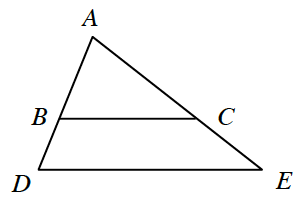### Home > INT1 > Chapter 11 > Lesson 11.1.1 > Problem11-7

11-7.In the diagram at right, $ΔABC$ and $ΔADE$ are similar. If $AB=5$, $BD=4$, and $BC=7$, then what is $DE$?

Separate the triangles into two similar triangles.

What is the length of $AD$? Once you know this, since the triangles are similar, the ratios of corresponding sides are equal.
Remember to set up proportions.

$DE=12.6$ units